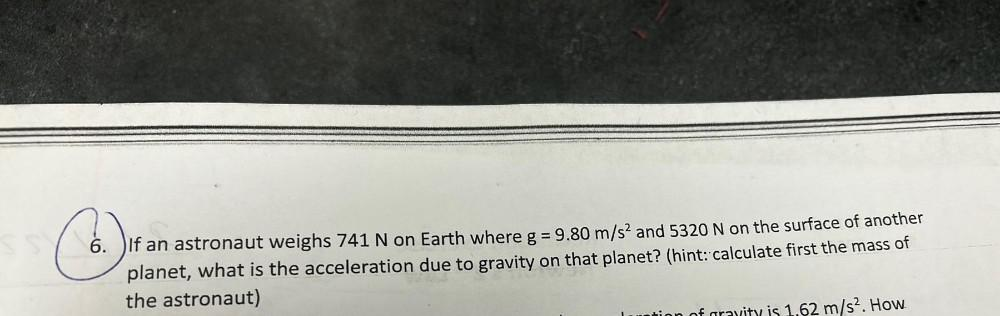Question:

# = 6. DIf an astronaut weighs 741 N on Earth where g = 9.80 m/s2 and 5320 N on the surface of another planet, what is the acceler= 6. DIf an astronaut weighs 741 N on Earth where g = 9.80 m/s2 and 5320 N on the surface of another planet, what is the acceleration due to gravity on that planet? (hint: calculate first the mass of the astronaut) m/s2. How